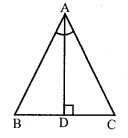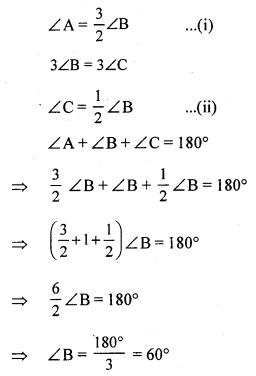## RS Aggarwal Class 7 Solutions Chapter 17 Constructions CCE Test Paper

These Solutions are part of RS Aggarwal Solutions Class 7. Here we have given RS Aggarwal Solutions Class 7 Chapter 17 Constructions CCE Test Paper.

Other Exercises

Question 1.
Solution:
Given, ∠ABO = 60°
∠CDO = 40°
⇒ ∠ABO = ∠BOC = 60° [alternate angles]Question 2.
Solution:
Here, AB || EC
∠BAC = ∠ACE = 70° (alternate angles)
⇒ ∠BCA = 180° – ∠BAC
⇒ ∠BCA = 180°- 120°
⇒ ∠BCA = 60°

Question 3.
Solution:
(i) ∠AOC = ∠BOD = 50° [vertically opposite angles]
(ii) ∠BOC = 180° – 50° (linear pair)
= 130°

Question 4.
Solution:
Here, 3x + 20 + 2x – 10 = 180
⇒ 5x + 10 = 180
⇒ 5x = 170
⇒ x = 34
∠AOC = (3 x 34 + 20)° = (102 + 20)° = 122°
∠BOC = (2 x 34 – 10)° = (68 – 10)° = 58°

Question 5.
Solution:
In ∆ABC, ∠A + ∠B + ∠C = 180°
⇒ 65° + 45° + ∠C= 180°
⇒ ∠C = 180° – 110° = 90°

Question 6.
Solution:
Let x = 2k and y = 3k
2k + 3k = 120° [Exterior angle property]
⇒ 5k = 120°
⇒ k = 24°
x = 2 x 24° = 48° and y = 3 x 24° = 72°
In ∆ABC :
∠A + ∠B + ∠C = 180°
⇒ 48° + 72° + ∠C = 180°
⇒ ∠C = 180°- 120°
⇒ ∠C = 60°
z = 60°

Question 7.
Solution:
Since it is a right triangle, by using the Pythagoras theorem:
Length of the hypotenuse = √(8² + 15²) = √(64 + 225) = √289 = ± 17 cm
The length of the side can not be negative.

Question 8.
Solution:
Given:
To show that ∆ABC is isosceles, we should show that ∠B = ∠C
∠BAD + ∠ABD = ∠DAC + ∠ACD (exterior angle property)
∠DAC + ∠ABD = ∠DAC + ∠ ACD [from equation (i)]
∠ABD = ∠ACD
This is because opposite angles of a triangle ∆ABC are equal.
Hence, ∆ABC is an isosceles triangle.Mark (✓) against the correct answer in each of the following :
Question 9.
Solution:
(c) 145°
The supplement of 35° = 180° – 35° = 145°

Question 10.
Solution:
(d) 124
x° + 56° = 180° (linear pair)
⇒ x = 180° – 56°
⇒ x = 124
x = 124°

Question 11.
Solution:
(c) 65°
∠ACD = 125°
∠ACD = ∠CAB + ∠ABC (the exterior angles are equal to the sum of its interior opposite angles)
∠ABC = 125° – 60° = 65°

Question 12.
Solution:
(c) 105°
∠A + ∠B + ∠C = 180°
⇒ ∠A = 180° – (40° + 35°)
⇒ ∠A = 105°

Question 13.
Solution:
(c) 60°
Given:
2∠A = 3∠BQuestion 14.
Solution:
(b) 55°
In ∆ABC :
A + B + C = 180° …(i)
Given, A – B = 33°
A = 33° + B …(ii)
B – C = 18°
C = B + 18° …(iii)
Putting the values of A and B in equation (i):
⇒ B + 33° + B + B – 18° = 180°
⇒ 3B = 180°
⇒ B = 55°

Question 15.
Solution:
(b) 3√2 cm
Here, AB = AC
In right angled isosceles triangle:
BC² = AB² + AC²
⇒ BC² = AB² + AB²
⇒ BC² = 2AB²
⇒ 36 = 2AB²
⇒ AB² = 18
⇒ AB = √18
⇒ AB = 3√2

Question 16.
Solution:
(i) The sum of the angles of a triangle is 180°.
(ii) The sum of any two sides of a triangle is always greater than the third side.
(iii) In ∆ABC, if ∠A = 90°, then BC² = (AB²) + (BC²)
(iv) In ∆ABC :
AB = AC
Then, BD = DC
This is because in an isosceles triangle, the perpendicular dropped from the vertex joining the equal sides, bisects the base.
(v) In the given figure, side BC of ∆ABC has produced to D and CE || BA.
If ∠ABC = 50°, then ∠ACE = 50°
AB || CE
∠BAC = ∠ACE = 50° (alternate angles)

Question 17.
Solution:
(i) True
(ii) True
(iii) False. Each acute angle of an isosceles right triangle measures 45°.
(iv) True.

Hope given RS Aggarwal Solutions Class 7 Chapter 17 Constructions CCE Test Paper are helpful to complete your math homework.

If you have any doubts, please comment below. Learn Insta try to provide online math tutoring for you.